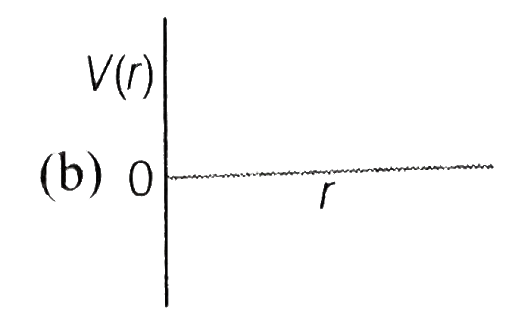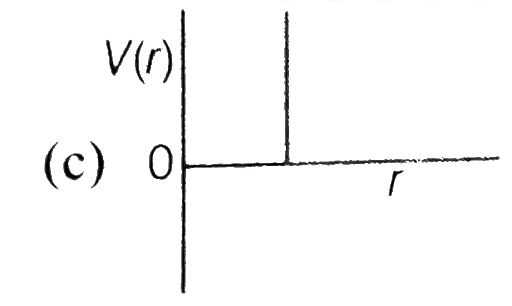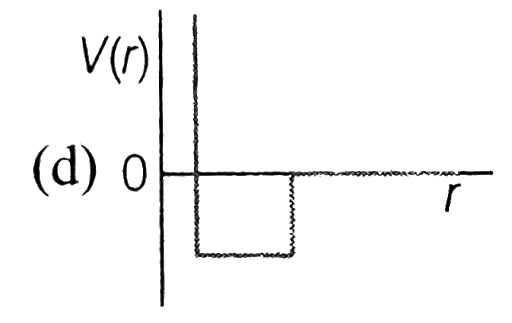One mole of a monoatomic real gas satisfies the equation p(V-b)=R | FiloClass 12

Chemistry

Solid State

States of Matter654

One mole of a monoatomic real gas satisfies the equation where is a constant. The relationship of interatomic potential and interatomic distance for gas is given by

1.2.3.4.654